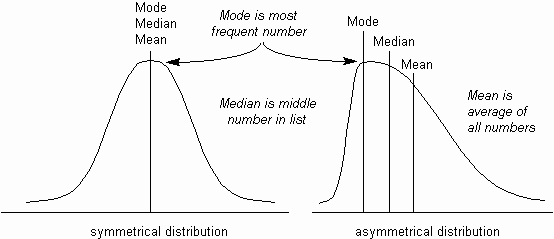The Psychology of Quality and More

# Measuring centering

The Quality Toolbook > Variation > Measuring centering

To measure the centering of a process requires that the center point of the set of results be identified. The accuracy of the process can then be determined by comparing it with target values. There are three ways of measuring this center point: the mean (or average), the median and the mode (see the figure below).Fig. 1. Mean, median and mode in distributions

## Mean

The most common way of measuring the center point of a set of measurements is with the average, or mean (i.e. the sum of all measurements divided by the total number of measurements).

The mean is useful for further mathematical treatment, as it considers all values (although a few extreme values can cause the mean to become unrepresentative of the rest of the values).

## Median

If the measurements are listed in numeric order, then the median is the number half-way down the list. If there is an even number of measurements, it is half-way between the middle two numbers. The median is not distorted by extreme values, but it can be very unrepresentative of the other values, particularly in a distribution which is not symmetrical.

## Mode

The mode is the most commonly occurring measurement. In a distribution graph, this is the highest point. The mode is also not distorted by extreme values, and is useful for measuring such as average earnings. However, there can be more than one mode, and it is not as good as the mean for mathematical treatment.

In a symmetrical distribution such as a Normal distribution, these three measures are the same. In an asymmetrical (or skewed) distribution, as below, there is a simple rule-of-thumb formula which can be used to estimate one, given the other two:

Mean - Mode = 3 x (Mean - Median)

### You can buy books here

And the big
paperback book